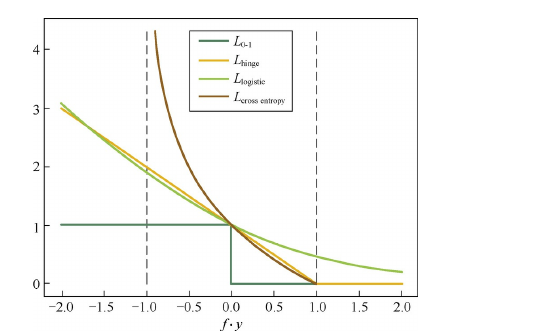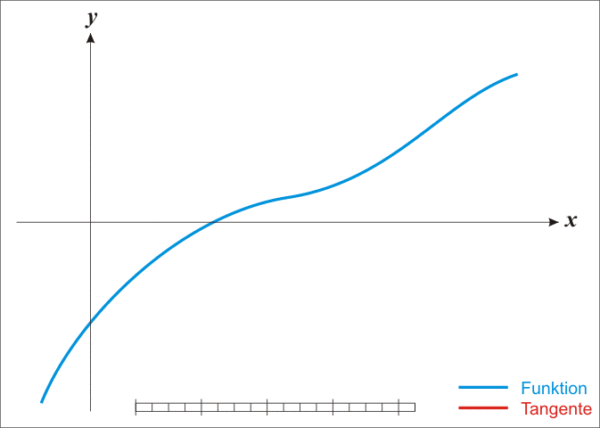# 优化算法

Posted by MaggicQ on April 13, 2019

# 常见优化算法

## 常见损失函数

### Hinge损失函数

Hinge损失在$fy\geq 1$处不可导，因此不能用梯度下降法进行优化，而是用次梯度下降法(Subgradient Descent Method)。

### 交叉熵损失函数## 梯度下降法

1. 取初值 $x^{\left(0\right)} \in R^{n}$ ，置 $k=0$
2. 计算 $f \left( x^{\left(k\right)}\right)$
3. 计算梯度 $g_{k}=g\left( x^{\left(k\right)} \right)$ ，当 $\| g_{k} \| < \varepsilon$ 时，停止迭代，令 $x^{*}=x^{\left(k\right)}$ ；否则，令 $p_{k}=-g\left(x^{\left(k\right)}\right)$ ，求 $\lambda_{k}$ ，使：

4. 置 $x^{\left(k+1\right)}= x^{\left(k\right)}+\lambda_{k}p_{k}$ ，计算 $f \left( x^{\left(k＋1\right)} \right)$ 当 $\| f \left( x^{\left(k＋1\right)} \right) - f \left( x^{\left(k\right)} \right) \| < \varepsilon$ 或 $\| x^{\left(k＋1\right)} - x^{\left(k\right)} \| < \varepsilon$ 时，停止迭代，令 $x^{*}=x^{k+1}$
5. 否则，置 $k=k+1$ ，转3.

1. 算法的步长选择。为了加快收敛速率，同时提高求解精度，通常会采用衰减学习速率的方案：一开始算法采用较大的学习速率，当误差曲线进入平台期后，减小学习速率做更精细的调整。最优的学习速率方案也通常需要调参才能得到。
2. 算法参数的初始值选择。初始值不同，获得的最小值也有可能不同，因此梯度下降求得的只是局部最小值；当然如果损失函数是凸函数则一定是最优解。由于有局部最优解的风险，需要多次用不同初始值运行算法，关键损失函数的最小值，选择损失函数最小化的初值。
3. 归一化。由于样本不同特征的取值范围不一样，可能导致迭代很慢，为了减少特征取值的影响，可以对特征数据归一化。
4. ​算法的batch size大小的选择，在不同的应用中，最优的batch size通常会不一样，需要通过调参选取。一般$m$取2的幂次时能充分利用矩阵运算操作，所以可以在2的幂次中挑选最优的取值，例如32、64、128、256等。

• 小批量梯度下降法（Mini-batch Gradient Descent）小批量梯度下降法是批量梯度下降法和随机梯度下降法的折衷，也就是对于 $m$ 个样本，我们采用 $x$ 个样子来迭代， $1<x<m$ 。一般可以取 $x=16,32,64…$ ，当然根据样本的数据，可以调整这个 $x$ 的值。

## 牛顿法和拟牛顿法

$\frac{f(x)-f(x_k)}{x-x_k} = f^{‘}(x_k) \Rightarrow f(x)=f(x_k) + f^{‘}(x_k) \cdot (x-x_k)$$f(x)$有极值的必要条件：它的一阶导在极值点处取值为0特别地，若是极小值点则 Hessian 是正定矩阵。 $f(x)$ 的一阶导为： $∇ f(x) = ∇ f(x_k) + H(x_k)(x-x_k)$ 因此，若从 $x_k$ 开始迭代，求 $f(x)$ 的极小点 $x_{k+1}$作为第 $k+1$ 次的迭代值。即：

1) 随机选择初始点 $x_0$ ，迭代次数 $k=0$ ；

2) 计算目标函数 $f(x)$ 在点 $x_k$ 的梯度 $g(x_k)$ 和Hessian 矩阵 $H(x_k)$； 若 $||g(x_k)|| <\epsilon$ ，停止计算，得到近似解： $x^\star=x(k)$

3) 根据迭代公式 $x_{k+1} = x_k - H^{-1}(x_k) ∇ f(x_k)$ 更新 $x$ 值；

## EM算法

EM算法也称期望最大化（Expectation-Maximum,简称EM）算法，它是一种迭代算法，用于含有隐变量（hidden variable）的概率模型参数的极大似然估计。

### EM算法的引入

EM算法的思路是使用启发式的迭代方法，既然我们无法直接求出模型分布参数，那么我们可以先猜想隐含数据（EM算法的E步），接着基于观察数据和猜测的隐含数据一起来极大化对数似然，求解我们的模型参数（EM算法的M步)。由于我们之前的隐藏数据是猜测的，所以此时得到的模型参数一般还不是我们想要的结果。不过没关系，我们基于当前得到的模型参数，继续猜测隐含数据（EM算法的E步），然后继续极大化对数似然，求解我们的模型参数（EM算法的M步)。以此类推，不断的迭代下去，直到模型分布参数基本无变化，算法收敛，找到合适的模型参数。

### EM算法的推导

EM算法是通过迭代逐步近似极大化$L(\theta)$的。假设在第$i$次迭代后$\theta$的估计值是$\theta^{(i)}$。我们希望新的估计值$\theta$能使$L(\theta)$增加，即$L(\theta) > L(\theta^{(i)})$，并逐步达到极大值。为此，考虑两者的差：

### EM算法的流程

（1）选择参数的初值$\theta^{(0)}$，开始迭代；

（2）E步：记$\theta^{(i)}$为第$i$次迭代参数$\theta$的估计值，在第$i+1$次迭代的E步，计算

​ 这里，$P(Z|Y, \theta^{(i)})$是在给定观测数据Y和当前的参数估计$\theta^{(i)}$下隐变量数据Z的条件概率分布；

​ 这里的Q函数是完全数据的对数似然函数$logP(Y, Z | \theta)$关于在给定预测数据$Y$和当前参数$\theta^{(i)}$下对未观测数据$Z$的条件概率分布$P(Z | Y, \theta^{(i)})$的期望

（3）M步：求使$Q(\theta, \theta^{(i)})$极大化的$\theta$，确定第$i+1$迭代次的参数的估计值$\theta^{(i+1)}$

（4）重复第2步和第3步，直到收敛。

• 参数的初值可以任意选择,但需注意EM算法对初值是敏感的。
• 每次迭代实际在求Q函数及其极大
• 迭代停止的条件一般是Q函数不再增长或者增长很小
• EM算法不能保证找到全局最优

## FAQ

### 无约束优化问题的优化方法有哪些?

• 直接法，就是能够直接给出优化问题最优解的方法。但是需要目标函数满足两个条件：

1. $L(\cdot)$是凸函数
2. $\nabla L\left(\theta^{*}\right)=0$ 有闭式解

这两个条件限制了它的应用范围，因此，在很多实际问题中，我们往往采用迭代法。

• ​迭代法就是迭代地修正对最优解的估计。迭代法又可以分为一阶法（梯度下降法）和二阶法（牛顿法和拟牛顿法）两类。二阶法的收敛速度一般要远快于一阶法，但是在高维情况下，$Hessian$矩阵求逆的计算复杂度很大，而且当目标函数非凸时，二阶法有可能会收敛到鞍点(Saddle Point)。

### 针对随机梯度下降法的缺点，目前有哪些优化的变种方法？RMSProp方法

$Adam$方法将惯性保持和环境感知这两个优点集于一身。一方面，$Adam$记录梯度的一阶矩(first moment)，即过往梯度与当前梯度的平均，这体现了惯性保持；另一方面，$Adam$还记录梯度的二阶矩(second moment)，即过往梯度平方与当前梯度平方的平均，这类似$AdaGrad$方法，体现了环境感知能力，为不同参数产一阶矩和二阶矩采用类似于滑动窗口内求平均的思想进行融合，即当前梯度和近一段时间内梯度的平均值，时间久远的梯度对当前平均值的贡献呈指数衰减(exponential decay average)。具体来说，一阶矩和二阶矩采用指数衰退平均技术，计算公式为：